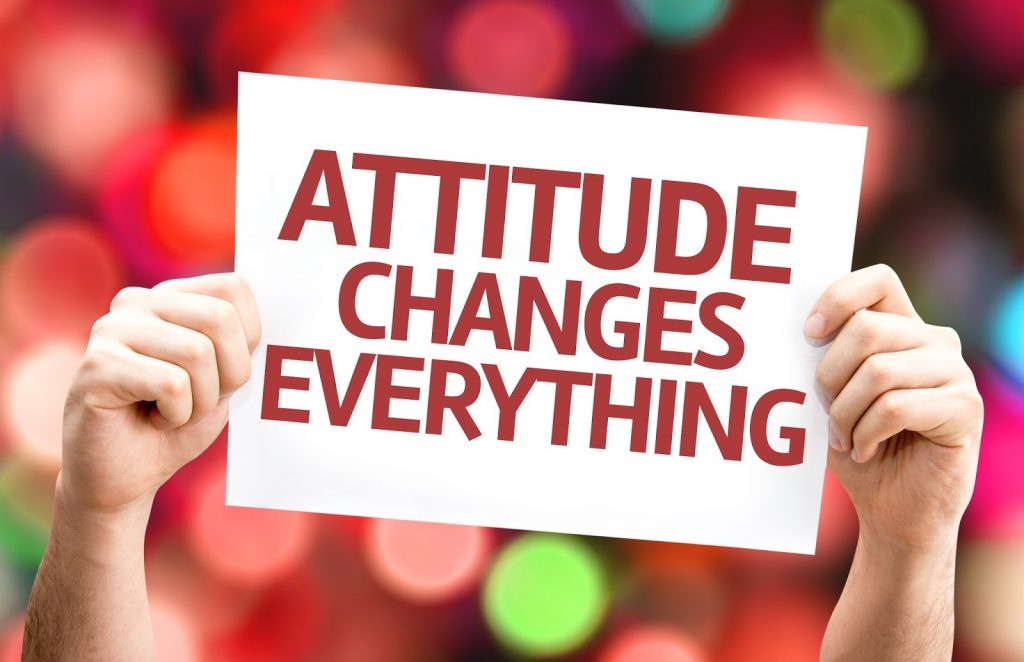Sign inEducation

College Education

# Money, Education, Love, Luck And Knowledge Can Never Solve Our Problems In Life But Rather Attitude

How to solve problems in life using alphabets and numbers."Life is a war" as many people used to say in their daily activities. People always resort to different ways and means to get their problem solved when they are in times of difficulties. You will be very amazed to see how this alphabets and numbers can help you solve any problem that comes across your way.

First of all let start by assigning a number to each of the alphabets. IF;

A = 1

B = 2

C = 3

D = 4

E = 5

F = 6

G = 7

H = 8

I = 9

J = 10

K = 11

L = 12

M = 13

N = 14

O = 15

P = 16

Q = 17

R = 18

S = 19

T = 20

U = 21

V = 22

W = 23

X = 24

Y = 25 and Z = 26.

Then let see if the following words can help us solve our daily problems. Hard work, knowledge, love, luck.

✓. H+A+R+D+W+O+R+K

8+1+18+4+23+15+18+11 = 98%

✓. K+N+O+W+L+E+D+G+E

11+14+15+23+12+5+4+7+5 = 96%

✓. L+O+V+E

12+15+22+5 = 54%

✓. L+U+C+K

12+21+3+11 = 47%

We can realised that none of them makes 100%. Then what makes 100%? Is it Money? NO!

✓. M+O+N+E+Y

13+15+14+5+25 = 72%

✓. E+D+U+C+A+T+I+O+N

5+4+21+3+1+20+9+15+14 = 92%

NO!

✓. L+E+A+D+E+R+S+H+I+P

12+5+1+4+5+18+19+8+9+16 = 97%

We can see from the above that education, hard work, love, luck leadership and money can't help us to solve our daily life problems. But every problem has a solution, only if we can only change our attitude.✓. ATTITUDE

A+T+T+I+T+U+D+E = 1+20+20+9+20+21+4+5 = 100%

It is therefore OUR ATTITUDE towards Life and work that makes OUR Life 100% Successful. Amazing mathematics, Let's change our Attitude of doing things in life.

Thank you for reading this post. Don't forget to circle back to the extreme right hand side corner of this post to click on the + follow button to follow up on this page for more interesting post like this and many more. Drop a like and comment for this page if you found this post very interesting. Be a blessing to someone today by sharing this post to as many as you can.

Content created and supplied by: Randasty (via Opera News )